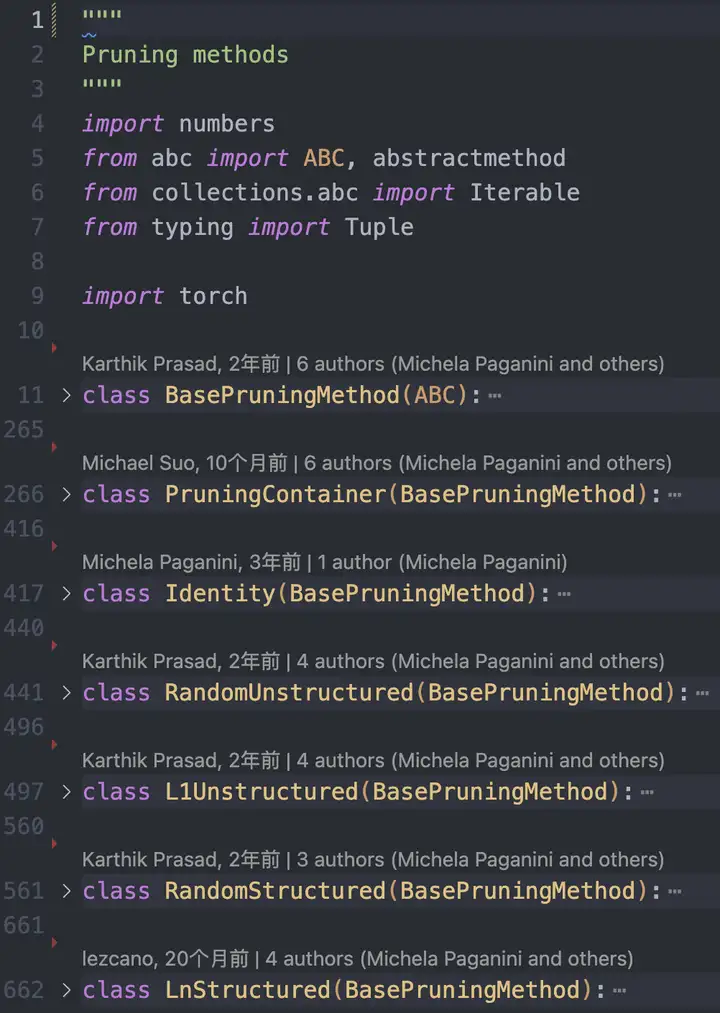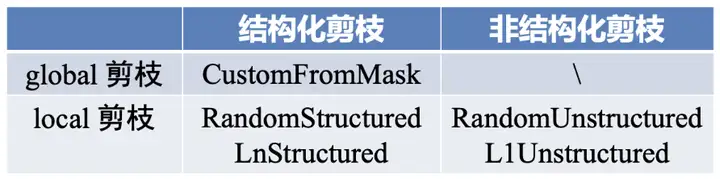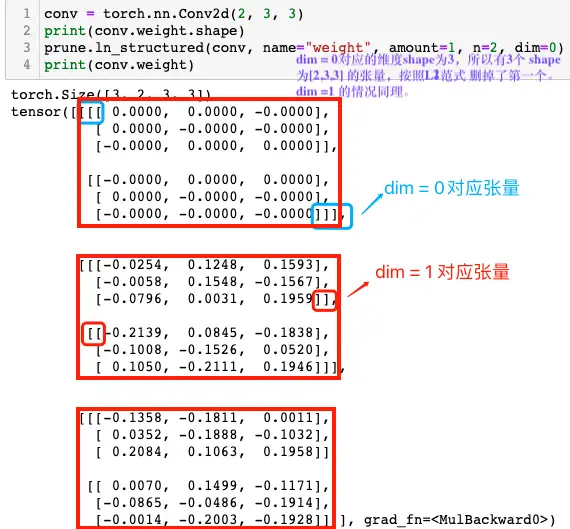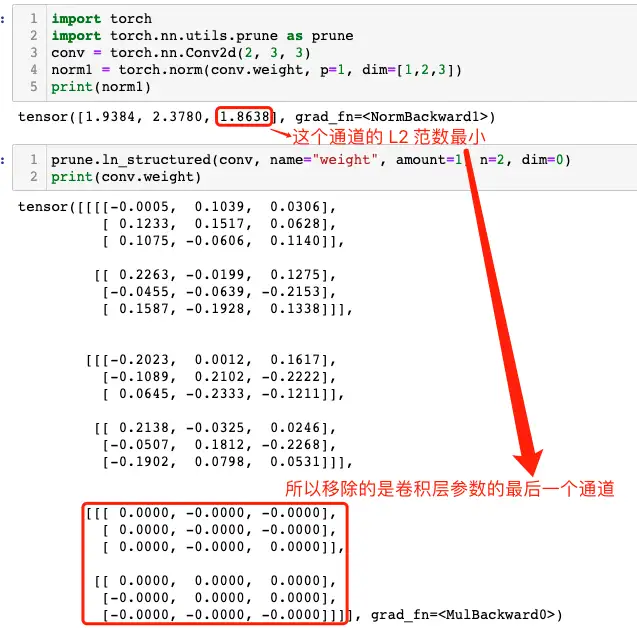# 实践Pytorch中的模型剪枝方法

## 二，PyTorch 的剪枝

• Random: 简单地修剪随机参数。
• Magnitude: 修剪权重最小的参数（例如它们的 L2 范数）

### 2.1，pytorch 剪枝工作原理Pytorch 将原始参数 <param> 复制到名为 <param>_original 的参数中，并创建一个缓冲区来存储剪枝掩码 <param>_mask。同时，其也会创建一个模块级的 forward_pre_hook 回调函数（在模型前向传播之前会被调用的回调函数），将剪枝掩码应用于原始权重。

pytorch 剪枝的 api 和教程比较混乱，我个人将做了如下表格，希望能将 api 和剪枝方法及分类总结好。pytorch 中进行模型剪枝的工作流程如下：

1. 选择剪枝方法（或者子类化 BasePruningMethod 实现自己的剪枝方法）。
2. 指定剪枝模块和参数名称。
3. 设置剪枝方法的参数，比如剪枝比例等。

### 2.2，局部剪枝

Pytorch 框架中的局部剪枝有非结构化和结构化剪枝两种类型，值得注意的是结构化剪枝只支持局部不支持全局。

#### 2.2.1，局部非结构化剪枝

1，局部非结构化剪枝（Locall Unstructured Pruning）对应函数原型如下：

``def random_unstructured(module, name, amount)``

1）函数功能

2）函数参数定义：

• module (nn.Module): 需要剪枝的网络层/模块，例如 nn.Conv2d() 和 nn.Linear()。
• name(str): 要剪枝的参数名称，比如 “weight” 或 “bias”。
• amount (int or float): 指定要剪枝的数量，如果是 0~1 之间的小数，则表示剪枝比例；如果是证书，则直接剪去参数的绝对数量。比如amount=0.2 ，表示将随机选择 20% 的元素进行剪枝。

3）下面是 random_unstructured 函数的使用示例。

``````import torch
import torch.nn.utils.prune as prune
conv = torch.nn.Conv2d(1, 1, 4)
prune.random_unstructured(conv, name="weight", amount=0.5)
conv.weight
"""
tensor([[[[-0.1703,  0.0000, -0.0000,  0.0690],
[ 0.1411,  0.0000, -0.0000, -0.1031],
[-0.0527,  0.0000,  0.0640,  0.1666],
[ 0.0000, -0.0000, -0.0000,  0.2281]]]], grad_fn=<MulBackward0>)
"""``````

#### 2.2.2，局部结构化剪枝

``````def random_structured(module, name, amount, dim)
def ln_structured(module, name, amount, n, dim, importance_scores=None)``````

1）函数功能

2）参数定义

n 表示剪枝的范数，dim 表示剪枝的维度。

• dim = 0： 移除一个神经元。
• dim = 1：移除与一个输入的所有连接。

• dim = 0(Channels) : 通道 channels 剪枝/过滤器 filters 剪枝
• dim = 1（Neurons）: 二维卷积核 kernel 剪枝，即与输入通道相连接的 kernel

#### 2.2.3，局部结构化剪枝示例代码

``class torch.nn.Conv2d(in_channels, out_channels, kernel_size, stride=1, padding=0, dilation=1, groups=1, bias=True)````````conv = torch.nn.Conv2d(2, 3, 3)
norm1 = torch.norm(conv.weight, p=1, dim=[1,2,3])
print(norm1)
"""
"""
prune.ln_structured(conv, name="weight", amount=1, n=2, dim=0)
print(conv.weight)
"""
tensor([[[[-0.0005,  0.1039,  0.0306],
[ 0.1233,  0.1517,  0.0628],
[ 0.1075, -0.0606,  0.1140]],
[[ 0.2263, -0.0199,  0.1275],
[-0.0455, -0.0639, -0.2153],
[ 0.1587, -0.1928,  0.1338]]],
[[[-0.2023,  0.0012,  0.1617],
[-0.1089,  0.2102, -0.2222],
[ 0.0645, -0.2333, -0.1211]],
[[ 0.2138, -0.0325,  0.0246],
[-0.0507,  0.1812, -0.2268],
[-0.1902,  0.0798,  0.0531]]],
[[[ 0.0000, -0.0000, -0.0000],
[ 0.0000, -0.0000, -0.0000],
[ 0.0000, -0.0000,  0.0000]],
[[ 0.0000,  0.0000,  0.0000],
[-0.0000,  0.0000,  0.0000],
"""``````dim = 1 的情况：

``````conv = torch.nn.Conv2d(2, 3, 3)
norm1 = torch.norm(conv.weight, p=1, dim=[0, 2,3])
print(norm1)
"""
"""
prune.ln_structured(conv, name="weight", amount=1, n=2, dim=1)
print(conv.weight)
"""
tensor([[[[ 0.0000, -0.0000, -0.0000],
[-0.0000,  0.0000,  0.0000],
[-0.0000,  0.0000, -0.0000]],
[[-0.2140,  0.1038,  0.1660],
[ 0.1265, -0.1650, -0.2183],
[-0.0680,  0.2280,  0.2128]]],
[[[-0.0000,  0.0000,  0.0000],
[ 0.0000,  0.0000, -0.0000],
[-0.0000, -0.0000, -0.0000]],
[[-0.2087,  0.1275,  0.0228],
[-0.1888, -0.1345,  0.1826],
[-0.2312, -0.1456, -0.1085]]],
[[[-0.0000,  0.0000,  0.0000],
[ 0.0000, -0.0000,  0.0000],
[ 0.0000, -0.0000,  0.0000]],
[[-0.0891,  0.0946, -0.1724],
[-0.2068,  0.0823,  0.0272],
"""``````

### 2.3，全局非结构化剪枝

``````# v1.4.0 版本
def global_unstructured(parameters, pruning_method, **kwargs)
# v2.0.0-rc2版本
def global_unstructured(parameters, pruning_method, importance_scores=None, **kwargs):``````

1）函数功能

2）参数定义

• parameters（(Iterable of (module, name) tuples)）: 修剪模型的参数列表，列表中的元素是 (module, name)。
• pruning_method（function）: 目前好像官方只支持 pruning_method=prune.L1Unstuctured，另外也可以是自己实现的非结构化剪枝方法函数。
• importance_scores: 表示每个参数的重要性得分，如果为 None，则使用默认得分。
• **kwargs: 表示传递给特定剪枝方法的额外参数。比如 amount 指定要剪枝的数量。

3）global_unstructured 函数的示例代码如下所示。

``````device = torch.device("cuda" if torch.cuda.is_available() else "cpu")
class LeNet(nn.Module):
def __init__(self):
super(LeNet, self).__init__()
# 1 input image channel, 6 output channels, 3x3 square conv kernel
self.conv1 = nn.Conv2d(1, 6, 3)
self.conv2 = nn.Conv2d(6, 16, 3)
self.fc1 = nn.Linear(16 * 5 * 5, 120) # 5x5 image dimension
self.fc2 = nn.Linear(120, 84)
self.fc3 = nn.Linear(84, 10)
def forward(self, x):
x = F.max_pool2d(F.relu(self.conv1(x)), (2, 2))
x = F.max_pool2d(F.relu(self.conv2(x)), 2)
x = x.view(-1, int(x.nelement() / x.shape))
x = F.relu(self.fc1(x))
x = F.relu(self.fc2(x))
x = self.fc3(x)
return x
model = LeNet().to(device=device)
model = LeNet()
parameters_to_prune = (
(model.conv1, 'weight'),
(model.conv2, 'weight'),
(model.fc1, 'weight'),
(model.fc2, 'weight'),
(model.fc3, 'weight'),
)
prune.global_unstructured(
parameters_to_prune,
pruning_method=prune.L1Unstructured,
amount=0.2,
)
# 计算卷积层和整个模型的稀疏度
# 其实调用的是 Tensor.numel 内内函数，返回输入张量中元素的总数
print(
"Sparsity in conv1.weight: {:.2f}%".format(
100. * float(torch.sum(model.conv1.weight == 0))
/ float(model.conv1.weight.nelement())
)
)
print(
"Global sparsity: {:.2f}%".format(
100. * float(
torch.sum(model.conv1.weight == 0)
+ torch.sum(model.conv2.weight == 0)
+ torch.sum(model.fc1.weight == 0)
+ torch.sum(model.fc2.weight == 0)
+ torch.sum(model.fc3.weight == 0)
)
/ float(
model.conv1.weight.nelement()
+ model.conv2.weight.nelement()
+ model.fc1.weight.nelement()
+ model.fc2.weight.nelement()
+ model.fc3.weight.nelement()
)
)
)
# 程序运行结果
"""
Sparsity in conv1.weight: 3.70%
Global sparsity: 20.00%
"""``````

## 三，总结

1. torch.nn.utils.prune.is_pruned(module): 判断模块 是否被剪枝。
2. torch.nn.utils.prune.remove(module, name)： 用于将指定模块中指定参数上的剪枝操作移除，从而恢复该参数的原始形状和数值。

## 参考资料

1. How to Prune Neural Networks with PyTorch
2. PRUNING TUTORIAL
3. PyTorch Pruning

「其他文章」